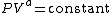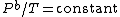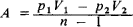Polytropic Process

Also found in: Medical, Wikipedia.

Polytropic process

A process which occurs with an interchange of both heat and work between the system and its surroundings. The nonadiabatic expansion or compression of a fluid is an example of a polytropic process. The interrelationships between the pressure (P) and volume (V) and pressure and temperature (T) for a gas undergoing a polytropic process are given by Eqs. (1) and

(1)(2)(2), where a and b are the polytropic constants for the process of interest. These constants, which are usually determined from experiment, depend upon the equation of state of the gas, the amount of heat transferred, and the extent of irreversibility in the process. See Isentropic process, Isothermal process, Thermodynamic processes

McGraw-Hill Concise Encyclopedia of Physics. © 2002 by The McGraw-Hill Companies, Inc.
The following article is from The Great Soviet Encyclopedia (1979). It might be outdated or ideologically biased.

Polytropic Process

a change in the state of a physical system where the system’s specific heat C remains constant. The curve representing a polytropic process on a thermodynamic diagram is called a polytropic curve.

A simple example of a reversible polytropic process is the polytropic change of an ideal gas. This process is defined by the equation pVn= const, where p is the pressure of the gas, V is the volume of the gas, and n = (C— CP)/(C— Cv) is the polytropic exponent (Cp and Cv are the specific heats of the gas at constant pressure and constant volume, respectively). By using the equation of state for an ideal gas, the equation of a polytropic curve can be written in a different form: pTn/(1-n)= const or VT1(1-n) = const, here T is the absolute temperature. Special cases of the equation of a polytropic process for an ideal gas are the equations for an isentropic curve, where C = 0 and n = Cp/cv (this ratio of specific heats is designated γ); an isobar, where C— Cp and n = 0; an isochor, where C= Cv and n = ∞; and an isotherm, where C= ∞ and n = 1. The work A done by an ideal gas against the ambient pressure is determined from the formulawhere the subscripts 1 and 2 refer to the initial and final states of the gas.

Engineering thermodynamics makes extensive use of the concept of polytropic processes in investigating the operating cycles of heat engines.